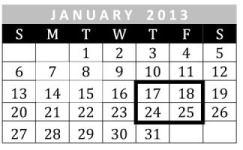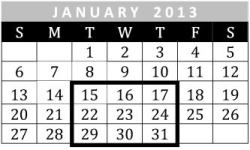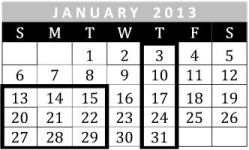## Tuesday, 8 January 2013

### Exploring Calendars in Primary Maths

Happy New Year!

The start of a new year sees the annual taking down and putting up one of the most frequently referred to data handling charts – the calendar.

Exploring calendars with your children provides lots of opportunities to link maths with other areas of the curriculum, particularly science, history and geography. Make use of this valuable opportunity by asking children to investigate some of the following.

Key Stage 1
- What patterns do you notice in the month of January? Are these patterns the same for February? What patterns are similar in these two months? What patterns are different?
- What similarities are there between the 2012 calendar and the 2013 calendar? What differences are there?- Choose any two by two square of dates from a month on a calendar. Add together the two pairs of numbers that are diagonally opposite, for example 7 + 15 and 8 + 14. What do you notice about the two answers? Repeat for other sets of four numbers. Try a different month. What do you notice? Explain why this happens.

Lower Key Stage 2
- In 2013, the Chinese New Year begins on February 10th.  According to the Chinese calendar, 2013 is the Year of the Snake. Investigate how the Chinese calendar works. Find a Chinese calendar and use it to work out which Chinese year you were born in. What about other members of your family?- Choose any two by two square of dates from a month on a calendar. Add the four numbers together. Multiply the smallest number by 4 and add 16 to the product. What do you notice about the two answers? Repeat for other sets of four numbers. Try a different month. What do you notice? Explain why this happens.- Choose any three by three square of dates from a month on a calendar. Add together the two pairs of numbers that are diagonally opposite, for example 15 + 31 and 17 + 29. What do you notice about the two answers? Compare these answers with the number in the centre of the square, i.e. 23. What do you notice? Repeat for other sets of nine numbers. Try a different month. What do you notice? Explain why this happens.

Upper Key Stage 2
Ask the children to investigate one or more of the following:
- January 1st marks the start of our Gregorian calendar. Investigate the history of the Gregorian calendar.
- Other calendars operate on a different cyclical pattern. Investigate other calendars such as Islamic, Jewish, Chinese or Hindu calendars.
- Investigate different historical calendars, for example, the Mayan, Egyptian, Sumerian, Athenian or Julian calendars.
- Our calendar year runs from January 1st until December 31st. Investigate other “years” such as the academic or fiscal years. How do these differ between countries?

- Choose any two by two square of dates from a month on a calendar. Multiply together the two pairs of numbers that are diagonally opposite, for example 9 x 17 and 10 x 16. What do you notice about the two answers? Repeat for other sets of four numbers. Try a different month. What do you notice? Explain why this happens.- Choose any three by three square of dates from a month on a calendar. Add together the numbers in the four corners, for example 13 + 15 + 27 + 29. Compare the sum with the number in the centre of the square, i.e. 21. What do you notice about the two numbers? Repeat for other sets of nine numbers. Try a different month. What do you notice? Explain why this happens.
- Choose any column of five dates from a month on a calendar. Add the five numbers together, for example 3 + 10 + 17 + 24 + 31. Compare the sum with the number in the middle of the column, i.e. 17. What do you notice about the two numbers? Repeat for other columns of five numbers. Try a different month. What do you notice? Explain why this happens.
- What other amazing patterns can you discover from a month on a calendar? Investigate dates in rectangles rather than squares, for example, 3 x 2 (or 2 x 3), 3 x 4 (or 4 x 3) and 4 x 2 (or 2 x 4) rectangles.

Peter Clarke
Series editor, Collins New Primary Maths